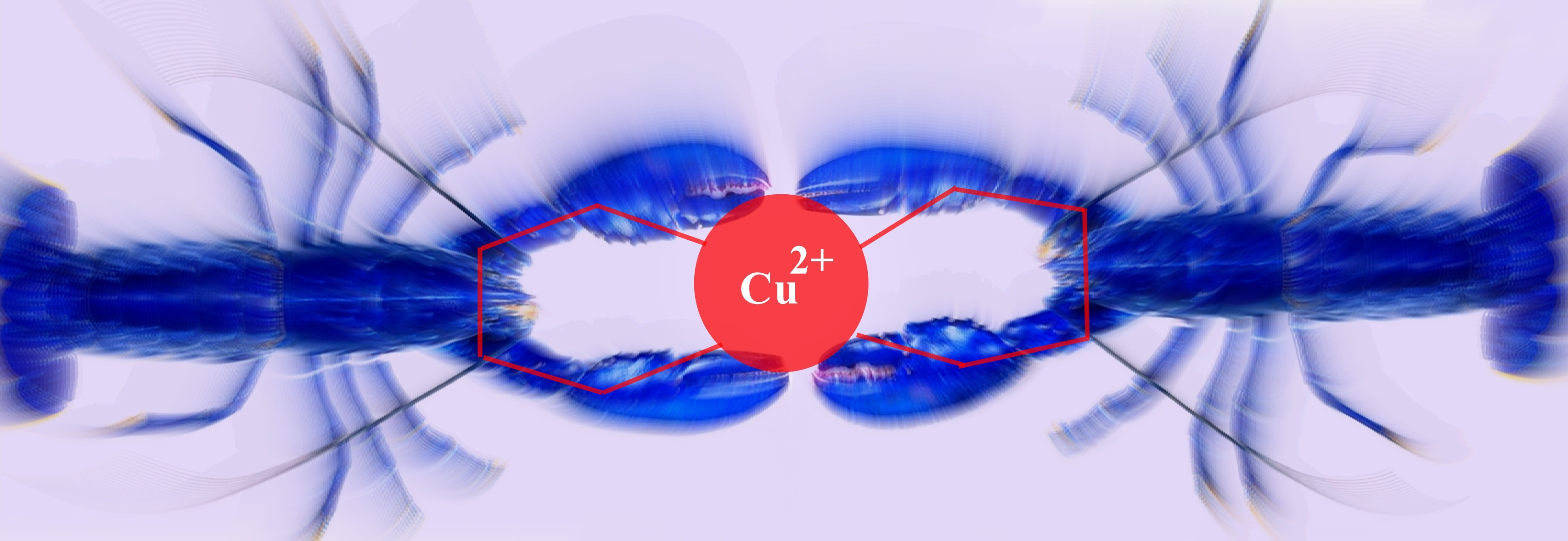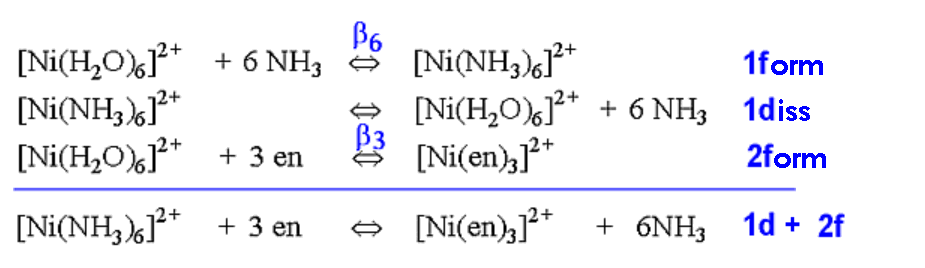## Stability, Chelation and the Chelate Effect

Lecture 5. CHEM1902 Coordination Chemistry

#### Introduction

A metal ion in solution does not exist in isolation, but in combination with ligands (such as solvent molecules or simple ions) or chelating groups, giving rise to complex ions or coordination compounds.

These complexes contain a central atom or ion, often a transition metal, and a cluster of ions or neutral molecules surrounding it. Many complexes are relatively unreactive species remaining unchanged throughout a sequence of chemical or physical operations and can often be isolated as stable solids or liquid compounds.

Other complexes have a much more transient existence and may exist only in solution or be highly reactive and easily converted to other species.

All metals form complexes, although the extent of formation and nature of these depend very largely on the electronic structure of the metal.

The concept of a metal complex originated in the work of Alfred Werner, who in 1913 was awarded the first Nobel Prize in Inorganic chemistry. A description of his life and the influence his work played in the development of coordination chemistry is given by G.B. Kauffman in "Inorganic Coordination Compounds", Heyden & Son Ltd, 1981.

Complexes may be non-ionic (neutral) or cationic or anionic, depending on the charges carried by the central metal ion and the coordinated groups. The total number of points of attachment to the central element is termed the coordination number and this can vary from 2 to greater than 12, but is usually 6.

The term ligand (ligare [Latin], to bind) was first used by Alfred Stock in 1916 in relation to silicon chemistry. The first use of the term in a British journal was by H. Irving and R.J.P. Williams in Nature, 1948, 162, 746 in their paper describing what is now called the Irving-Williams series.

For a fascinating review of the origin and dissemination of the term 'ligand' in chemistry see: W.H. Brock, K.A Jensen, C.K. Jorgensen and G.B. Kauffman, Polyhedron, 2, 1983, 1-7.

Ligands can be further characterised as monodentate, bidentate, tridentate etc. where the concept of teeth (dent) is introduced, hence the idea of bite angle etc.

The term chelate was first applied in 1920 by Sir Gilbert T. Morgan and H.D.K. Drew [J. Chem. Soc., 1920, 117, 1456], who stated:
"The adjective chelate, derived from the great claw or chela (from the Greek χηλη) of the lobster or other crustaceans, is suggested for the caliperlike groups which function as two associating units and fasten to the central atom so as to produce heterocyclic rings."Metal complexation is of widespread interest. It is studied not only by inorganic chemists, but by physical and organic chemists and by biochemists, pharmacologists, molecular biologists and environmentalists.

#### Thermodynamic Stability

In the laboratory course, it will have been pointed out that the "stability of a complex in solution" refers to the degree of association between the two species involved in the state of equilibrium. Qualitatively, the greater the association, the greater the stability of the compound. The magnitude of the (stability or formation) equilibrium constant for the association, quantitatively expresses the stability. Thus, if we have a reaction of the type:

M           +           4L           ⇄          ML4

then the larger the stability constant, the higher the proportion of ML4 that exists in the solution. Free metal ions rarely exist in solution so that M, will usually be surrounded by solvent molecules which will compete with the ligand molecules, L, and be successively replaced by them. For simplicity, we generally ignore these solvent molecules and write four stability constants as follows:

1. M + L ⇄ ML               K1 = [ML [L]] / [M]
2. ML + L ⇄ ML2           K2 = [ML2] / [ML] [L]
3. ML2 + L ⇄ ML3         K3 = [ML3] / [ML2] [L]
4. ML3 + L ⇄ ML4         K4 = [ML4] / [ML3] [L]

where K1, K2 etc. are referred to as "stepwise stability constants".
Alternatively, we can write the "Overall Stability Constant" denoted by βn thus:

M          +           4L          ⇄          ML4           β4 = [ML4]/ [M] [L]4

The stepwise and overall stability constants are therefore related as follows:

β4=K1.K2.K3.K4 or more generally,
βn=K1.K2.K3.K4--------------K n

Consider the four steps involved in the formation of the cuprammonium ion Cu(NH3)42+:

STEP 1:     Cu2++NH ⇄  Cu(NH3)2+

where K1   =     [Cu(NH3)2+]
[Cu2+][NH3]

STEP 2:     Cu(NH3)2+ + NH ⇄  Cu(NH3)2+2

where K2  =       [Cu(NH3)22+]
[Cu(NH3)2+][NH3]

STEP 3:     Cu(NH3)22+ + NH3  ⇄  Cu(NH3)32+

where K3  =       [Cu(NH3)32+]
[Cu(NH3)22+][NH3]

STEP 4:     Cu(NH3)32+ + NH3 ⇄  Cu(NH3)42+

where K4  =       [Cu(NH3)42+]
[Cu(NH3)32+][NH3]

where the {K} constants are the stepwise stability constants.
In terms of overall stability constant:

β4   =     [Cu(NH3)42+]
[Cu2+][NH3]4

The addition of the four ammine groups to copper shows a pattern found for most formation constants, in that the successive stability constants decrease. In this case, the four constants are:

logK1 =4.0, logK2 =3.2, logK3 =2.7, logK4 =2.0 or logβ4 =11.9

A number of texts refer to the instability constant or the dissociation constant of coordination complexes. This value corresponds to the reciprocal of the formation constant, since the reactions referred to are those where fully formed complexes break down to the aqua ion and free ligands.
This should be compared with the equation for the formation constant given earlier.

It is usual to represent the metal-binding process by a series of stepwise equilibria. This can lead to stability constants that may vary numerically from hundreds to enormous values such as 1035 and more.
That is 100,000,000,000,000,000,000,000,000,000,000,000.0
For this reason, they are commonly reported as logarithms.
so log10 (β) = log10 (1035) = 35.
For this reason it is useful to use logarithms, since log(K) is directly proportional to the free energy of the reaction.

ΔG = -RTln(β)
ΔG = -2.303 RTlog10(β)
ΔG = ΔH - TΔS

For a problem relating to metal complex formation and calculations of free metal ions concentrations, try your hand at CALCULATION # ONE. Other problems can be found in the Tutorial paper for this course.

#### The Chelate Effect

The chelate effect can be seen by comparing the reaction of a chelating ligand and a metal ion with the corresponding reaction involving comparable monodentate ligands. For example, comparison of the binding of 2,2'-bipyridine with pyridine or 1,2-diaminoethane (ethylenediamine=en) with ammonia.

It has been known for many years that a comparison of this type always shows that the complex resulting from coordination with the chelating ligand is much more thermodynamically stable. This can be seen by looking at the values for adding two monodentates compared with adding one bidentate, or adding four monodentates compared to two bidentates, or adding six monodentates compared to three bidentates.

#### Some tables of thermodynamic data

 # of ligands ΔG⦵ /kJmol-1 ΔH⦵ /kJmol-1 ΔS⦵ /JK-1mol-1 log β 2 NH3(1 en) -28.24 (-33.30) -29.79 (-29.41) -5.19 (+13.05) 4.95 (5.84) 4 NH3(2 en) -42.51 (-60.67) -53.14 (-56.48) -35.50 (+13.75) 7.44 (10.62)

 # of ligands log β ΔG⦵ /kJmol-1 2 py (1 bipy) 3.5 (6.9) -20 (-39) 4 py (2 bipy) 5.6 (13.6) -32 (-78) 6 py (3 bipy) 9.8 (19.3) -56 (-110)

 # of ligands log β ΔG⦵ /kJmol-1 1 NH3 2.8 -16 2 NH3 (1 en) 5.0 (7.51) -28.5 (-42.8) 3 NH3 6.6 -37.7 4 NH3 (2 en) 7.87 (13.86) -44.9 (-79.1) 5 NH3 8.6 -49.1 6 NH3 (3 en) 8.61 (18.28) -49.2 (-104.4)

A number of points can be highlighted from this data.
In the first table, it can be seen that the ΔH values for the formation steps are almost identical, that is, heat is evolved to about the same extent whether forming a complex involving monodentate ligands or bidentate ligands.
What is seen to vary significantly is the ΔS term which changes from negative (unfavourable) to positive (favourable). Note as well that there is a dramatic increase in the size of the ΔS term for adding two compared to adding four monodentate ligands. (-5 to -35 JK-1mol-1).
What does this imply, if we consider ΔS to give a measure of disorder?

In the case of complex formation of Ni2+ with ammonia or 1,2-diaminoethane, by rewriting the equilibria, the following equations are produced.Using the equilibrium constant for the reaction (3 above) where the three bidentates replace the six monodentates, it has been found that at a temperature of 25 °C (298 K):
ΔG = -2.303 RT log10 (K)
= -2.303 R T (18.28 - 8.61)
= -54 kJ mol-1
Based on measurements made over a range of temperatures, it is possible to break the ΔG term down into its enthalpy and entropy components. ΔG = ΔH - TΔS
The result is that:
ΔH = -29 kJ mol-1
- TΔS = -25 kJ mol-1
and at 25 °C (298 K)
ΔS = +88 J K-1 mol-1

Note that for many years, these numbers were incorrectly recorded in textbooks.
For example, the third edition of "Basic Inorganic Chemistry" by F.A. Cotton, G. Wilkinson and P.L. Gaus, John Wiley & Sons, Inc, 1995, on page 186 gave the values as:
ΔG = -67 kJ mol-1
ΔH = -12 kJ mol-1
- TΔS = -55 kJ mol-1
The conclusion they drew from these incorrect numbers was that the chelate effect was essentially an entropy effect, since the TΔS contribution was nearly 5 times bigger than that of ΔH.

In fact, the breakdown of the ΔG into ΔH and TΔS shows that the two terms are nearly equal (-29 cf. -25 kJ mol-1) with the ΔH term a little larger! The entropy term found is still much larger than for ordinary reactions involving substitution of a non-chelating ligand at a metal ion.
How can we explain this enhanced contribution from entropy? One explanation is to count the number of species on the left and right hand side of the equation above.
It will be seen that on the left-hand-side there are 4 species, whereas on the right-hand-side there are 7 species, that is a net gain of 3 species occurs as the reaction proceeds. This can account for the increase in entropy since it represents an increase in the disorder of the system.

An alternative view comes from trying to understand how the reactions might proceed. To form a complex with 6 monodentates requires 6 separate favourable collisions between the metal ion and the ligand molecules. To form the tris-bidentate metal complex requires an initial collision for the first ligand to attach by one arm but remember that the other arm is always going to be nearby and only requires a shift in position of the other end to enable the ligand to form the chelate ring.
If you consider dissociation steps, then when a monodentate group is displaced, it is lost into the bulk of the solution. On the other hand, if one end of a bidentate group is displaced the other arm is still attached and it is only a matter of the arm shifting around and it can be reattached again.

Both sets of conditions favour the formation of the complex with bidentate groups over that with monodentate groups.

For a problem relating to metal complex formation and calculations of thermodynamic values, try your hand at CALCULATION # TWO. Once again, further problems can be found in the Tutorial papers for this course.

To get a simplistic view of the replacement of ammonia groups by 1,2-diaminoethane try downloading a movie clip (1.7 MB, .avi format).
Note, for those linking from off-campus this may not be possible due to bandwidth and timeout problems!

See the ChemWIKI page on chelation or chelation at wikipedia.Return to Chemistry, UWI-Mona, Home Page